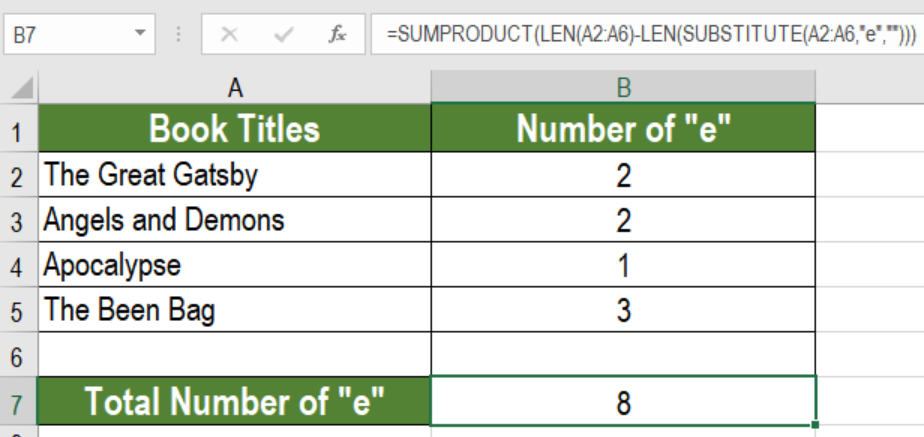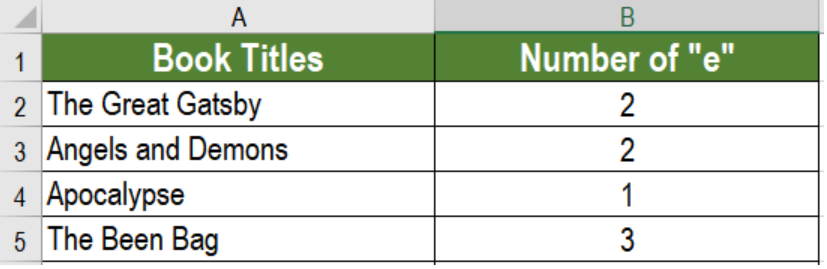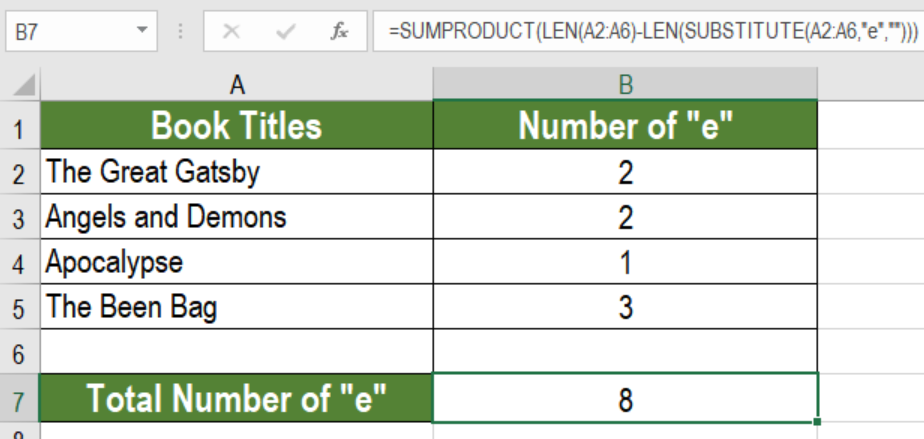Get instant live expert help with Excel or Google Sheets“My Excelchat expert helped me in less than 20 minutes, saving me what would have been 5 hours of work!”

#### Post your problem and you’ll get Expert help in seconds.

Your message must be at least 40 characters
Our professional Expert are available now. Your privacy is guaranteed.

# Count Specific Characters in a Range in Excel

Excel allows users to perform various tasks working with characters. We can count specific characters in range using SUMPRODUCT, SUBSTITUTE, and LEN functions. In this tutorial, we will learn how to count specific characters in a range in Excel.Figure 1. Example of how to Count Specific Characters in a range

## Generic Formula

`=SUMPRODUCT(LEN(range)-LEN(SUBSTITUTE(range,character,"")))`

## Process

Here, the SUBSTITUTE function removes all occurrences of the character to be counted. The LEN function counts the length of the text without the character to be counted. The previous value is subtracted from this length.

The SUMPRODUCT creates an array with one item per cell in the range. Each item contains a number from the above calculation. Next, SUMPRODUCT sums these numbers. Finally, it returns a total which represents the count of the character.

## Setting up Data

The following data contains some sample text. Column A contains these texts. Column B has the number of times “e” exist in these texts.Figure 2. The Sample Data

To count the total number of times ‘e’ exists in these texts, we need to:

• Go to cell B7. Click on it with the mouse.
• Assign the formula `=``SUMPRODUCT(LEN(A2:A6)-LEN(SUBSTITUTE(A2:A6,"g","")))` to B7.
• Press Enter.Figure 3. Applying the Formula

This will show the number of times column A contains the character ‘e’, which is 8.

## Notes

SUBSTITUTE is case-sensitive. It matches case performing a substitution. To count both lower and upper case occurrences, we need to nest the UPPER inside SUBSTITUTE. This will convert the text to uppercase. This, in turn will provide an uppercase character to count.

Most of the time, the problem you will need to solve will be more complex than a simple application of a formula or function. If you want to save hours of research and frustration, try our live Excelchat service! Our Excel Experts are available 24/7 to answer any Excel question you may have. We guarantee a connection within 30 seconds and a customized solution within 20 minutes.

### Did this post not answer your question? Get a solution from connecting with the expert.Another blog reader asked this question today on Excelchat:
Solution examplesI am trying to figure out how to use a sumif on SUBSTITUTE(A2,"*","~*") where A2 is a formula with 3371-4
Solved by M. Y. in 19 minsi have a table with Dates, First names , Last names and i want to count how many times a name occurs, but if a name occurs more than once on a particular date i need to only count it once. this is on excel and not google sheets
Solved by F. H. in 31 minsI need cell B2 Green if cells G2:Q2 are empty. If cells G2:Q2 have any text in any cell then i need B2 to be red.
Solved by C. W. in 20 minsHello, I need help with IF and SUBSTITUTE formula in excel
Solved by A. A. in 43 minsI have text and number in multiple cells (Ex: Cell 1: 4S, Cell 2: 3.5A, Cell 3: 8O) and want to sum the number values only (Ex: 4 + 3.5 + 8). How can I do this?
Solved by O. A. in 60 mins## Subscribe to Excelchat.coAnother blog reader asked this question today on Excelchat: